# Chapter 11 Trigonometric Identities 11 1 Trigonometric Identities

• Slides: 14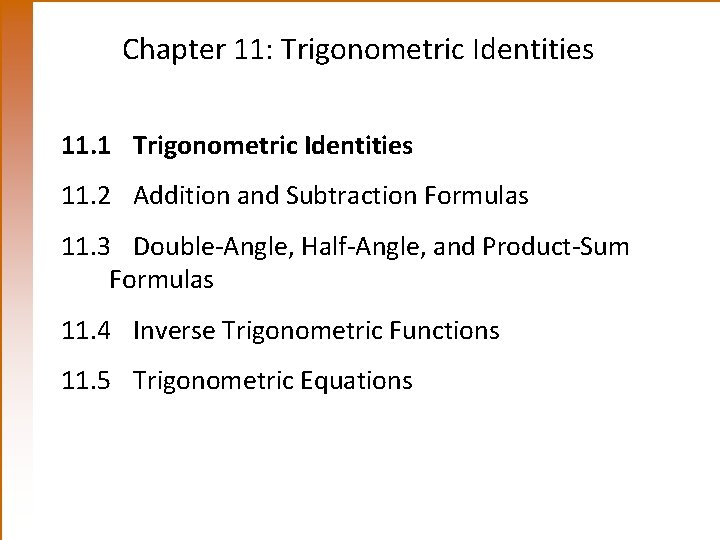Chapter 11: Trigonometric Identities 11. 1 Trigonometric Identities 11. 2 Addition and Subtraction Formulas 11. 3 Double-Angle, Half-Angle, and Product-Sum Formulas 11. 4 Inverse Trigonometric Functions 11. 5 Trigonometric Equations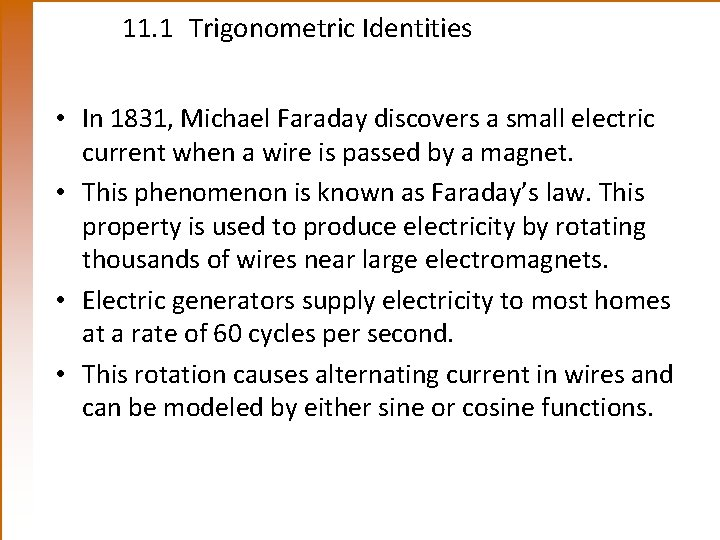11. 1 Trigonometric Identities • In 1831, Michael Faraday discovers a small electric current when a wire is passed by a magnet. • This phenomenon is known as Faraday’s law. This property is used to produce electricity by rotating thousands of wires near large electromagnets. • Electric generators supply electricity to most homes at a rate of 60 cycles per second. • This rotation causes alternating current in wires and can be modeled by either sine or cosine functions.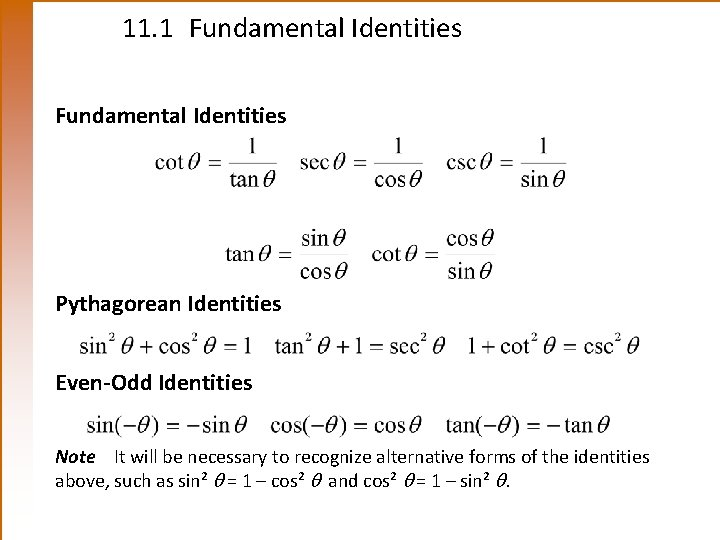11. 1 Fundamental Identities Pythagorean Identities Even-Odd Identities Note It will be necessary to recognize alternative forms of the identities above, such as sin² = 1 – cos² and cos² = 1 – sin² .11. 1 Fundamental Identities Cofunction Identities11. 1 Looking Ahead to Calculus • Work with identities to simplify an expression with the appropriate trigonometric substitutions. • For example, if x = 3 tan , then11. 1 Expressing One Function in Terms of Another Example Express cos x in terms of tan x. Solution Since sec x is related to both tan x and cos x by identities, start with tan² x + 1 = sec² x. Choose + or – sign, depending on the quadrant of x.11. 1 Rewriting an Expression in Terms of Sine and Cosine Example Write tan + cot in terms of sin and cos . Solution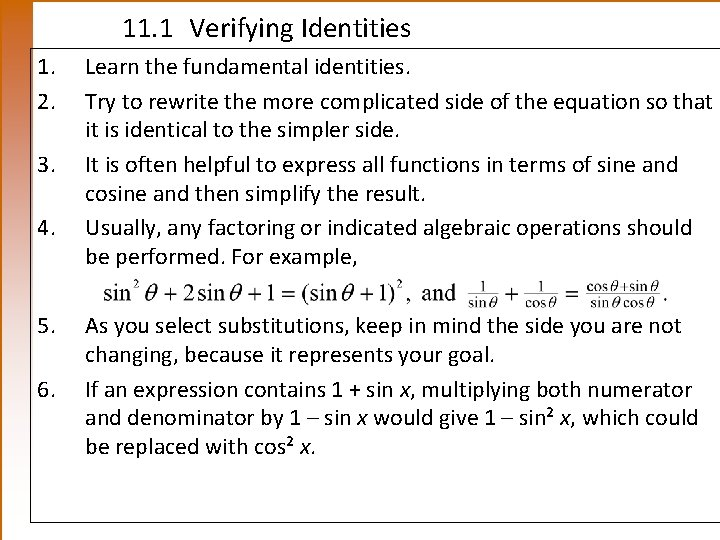11. 1 Verifying Identities 1. 2. 3. 4. 5. 6. Learn the fundamental identities. Try to rewrite the more complicated side of the equation so that it is identical to the simpler side. It is often helpful to express all functions in terms of sine and cosine and then simplify the result. Usually, any factoring or indicated algebraic operations should be performed. For example, As you select substitutions, keep in mind the side you are not changing, because it represents your goal. If an expression contains 1 + sin x, multiplying both numerator and denominator by 1 – sin x would give 1 – sin² x, which could be replaced with cos² x.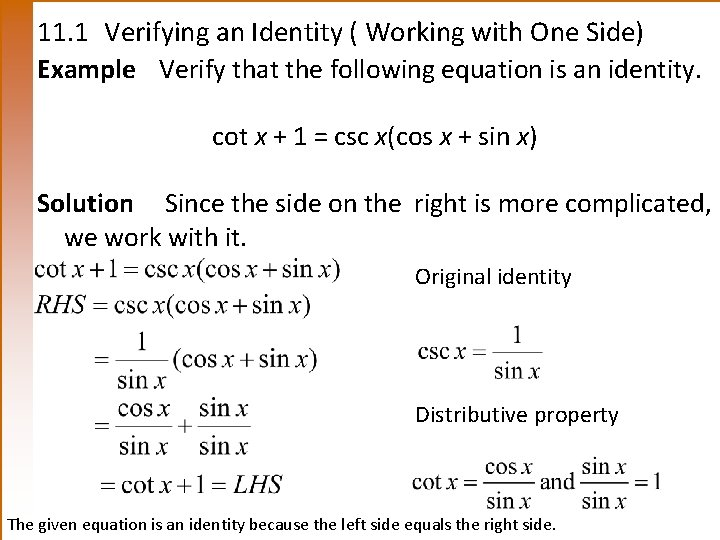11. 1 Verifying an Identity ( Working with One Side) Example Verify that the following equation is an identity. cot x + 1 = csc x(cos x + sin x) Solution Since the side on the right is more complicated, we work with it. Original identity Distributive property The given equation is an identity because the left side equals the right side.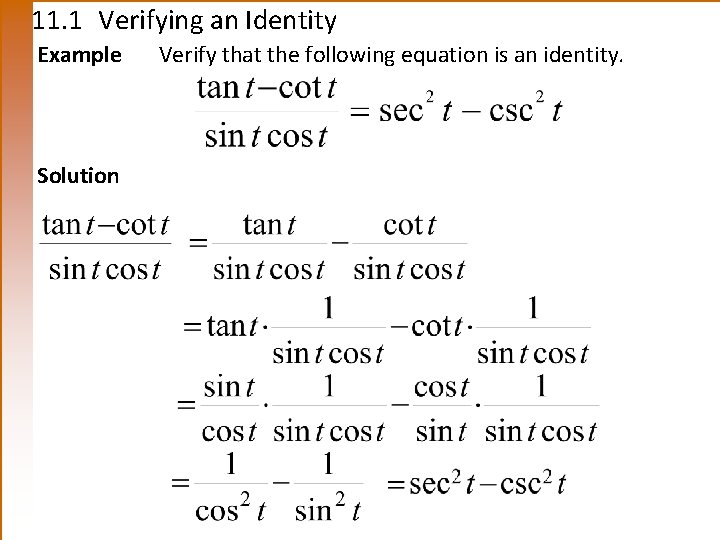11. 1 Verifying an Identity Example Solution Verify that the following equation is an identity.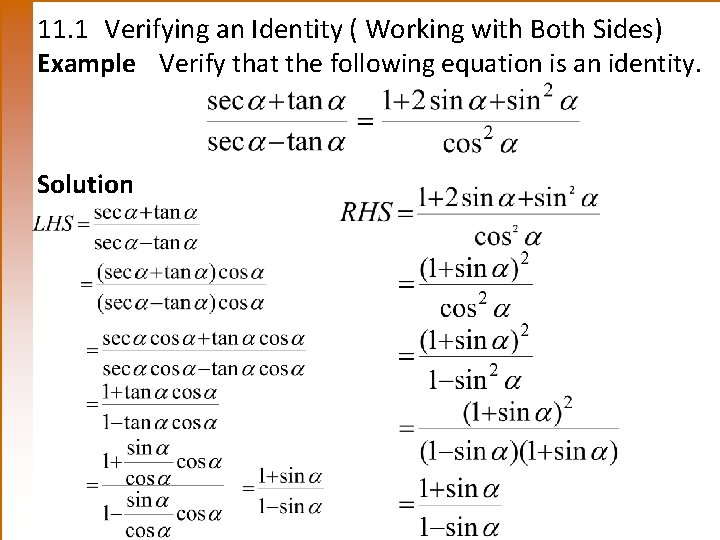11. 1 Verifying an Identity ( Working with Both Sides) Example Verify that the following equation is an identity. Solution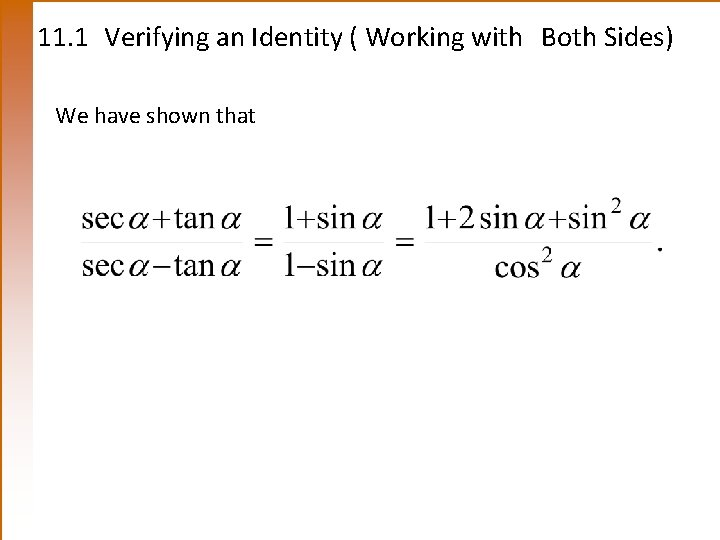11. 1 Verifying an Identity ( Working with Both Sides) We have shown that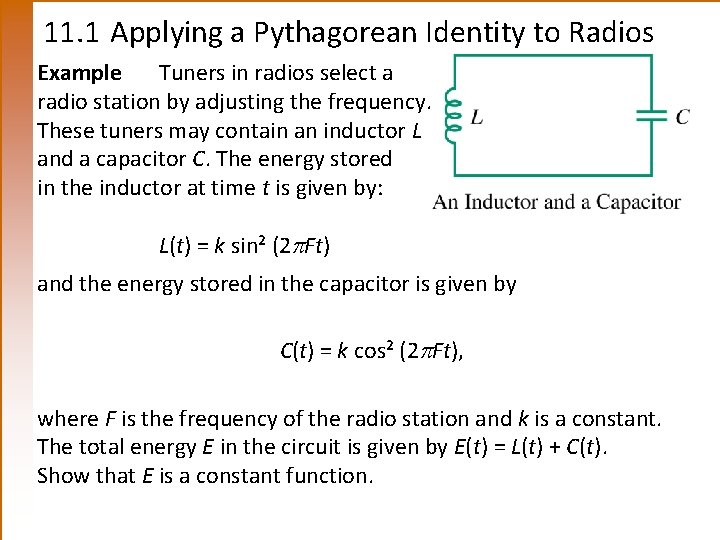11. 1 Applying a Pythagorean Identity to Radios Example Tuners in radios select a radio station by adjusting the frequency. These tuners may contain an inductor L and a capacitor C. The energy stored in the inductor at time t is given by: L(t) = k sin² (2 Ft) and the energy stored in the capacitor is given by C(t) = k cos² (2 Ft), where F is the frequency of the radio station and k is a constant. The total energy E in the circuit is given by E(t) = L(t) + C(t). Show that E is a constant function.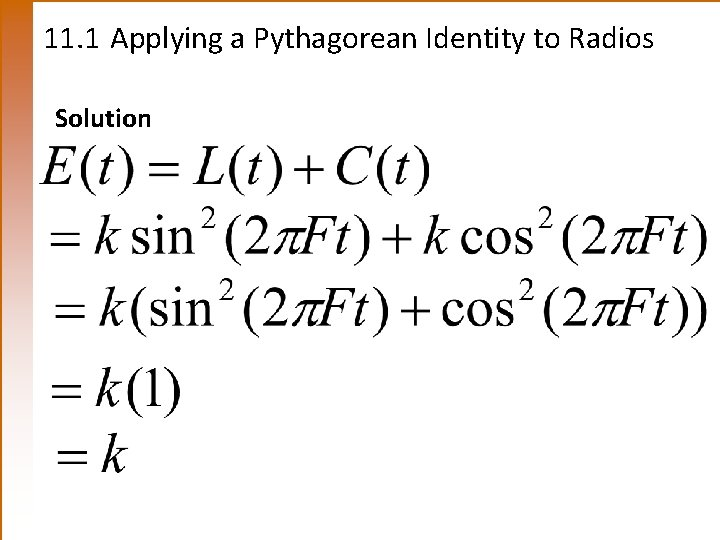11. 1 Applying a Pythagorean Identity to Radios Solution